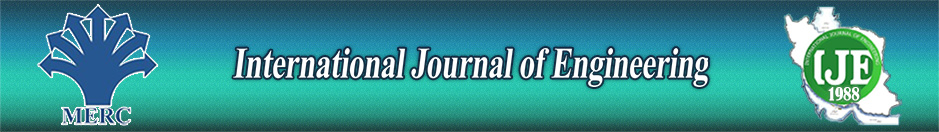Optimum Drill Bit Selection by Using Bit Images and Mathematical Investigation

Authors

1 Petroleum Engineeing, Universiti Teknologi PETROPNAS

2 Chemical Engineering, Oklahoma State University

3 Petroleum Geoscience, Universiti Teknologi PETROPNAS

Abstract

This study is designed to consider the two important yet often neglected factors, which are factory recommendation and bit features, in optimum bit selection. Image processing techniques have been used to consider the bit features. A mathematical equation, which is derived from a neural network model, is used for drill bit selection to obtain the bit’s maximum penetration rate that corresponds to the optimum parameters for drilling. At the end, the bit with the maximum penetration rate is chosen. The results of this study showed that bit pattern can be inserted in the calculation through a proper bit image processing technique. This is to ensure that each unique bit can be discriminated from other bits. The values of mean square error  and coefficient of determination (R2) were respectively found as  0.0037 and 0.9473, for the rate of penetration model. The image processing techniques were used to extract the bit features. The artificial neural network black box was converted to white box in order to extract a mathematical equation and visibility of the model.

Keywords